## Research Outputs

Most of my publications are available on arXiv and can also be accessed from MathSciNet. However, if for any reason, you can’t download them, send me an email and I will be happy to send you a copy.

# Publications

1. Diophantine approximation on curves, Preprint: arXiv:1902.02094 (with J. Schleischitz and D. Simmons).
2. Higher dimensional shrinking target problem in beta dynamical systems, Preprint:arXiv:1908.02098  (with Weiliang Wang).
3. Dynamical Borel-Cantelli lemma for recurrence theory, Preprint:arXiv:2009.03515(with B. Li, D. Simmons, and BW Wang) .
4. Metrical properties for continued fractions of formal Laurent series, Preprint:arXiv:2009.03513 (with H. Hu and Y. Yu).
5. The generalised Hausdorff measure of sets of Dirichlet non-improvable numbers,  Preprint:arXiv:2010.14760  (with P. Bos and D. Simmons)
6. Layered interference alignment: total DOF of MIMO X-channels, Preprint:arXiv:1412.7188. (with S. H. Mahboubi, A.S. Motahari and A. K. Khandani).
7. Generalised Baker-Schmidt Problem for hypersurfaces. In Press: International Mathematical Research Notices (IMRN). Pre- Print:arXiv:1803.02314v1.(with Johannes Schleischitz and David Simmons).
8. The sets of Dirichlet non-improvable numbers vs well-approximable numbers. Ergodic Theory Dynam. Systems 40 (2020), no. 12, 3217–3235. (with A. Bakhtawar and P. Bos)
9. Hausdorff dimension of an exceptional set in the theory of continued fractions.  Nonlinearity 33 (2020) 2615–2639. (with A. Bakhtawar and P. Bos).
10. Metrical theorems on systems of small affine forms.  Journal of Number Theory 2013 (2020) 67–100. (with S. Kristensen and David Simmons).
11. Metrical results for small linear forms and applications in signal processing. From Analysis to Visualization, Springer Proceedings in Mathematics & Statistics, vol 313 (2020) pp 377–393.
(with S. H. Mahboubi and A.S. Motahari).
12. A general principle for Hausdorff measure. Proc. Amer. Math. Soc. 147 (2019), no. 9, 3897–3904. (with David Simmons).
13. Hausdorff measure of sets of Dirichlet non-improvable numbers. Mathematika 64 (2018) 502–518 (with D. Kleinbock, N. Wadleigh, B.W. Wang).
14. A problem in non-linear Diophantine approximation. Nonlinearity 31 (2018) 1734–1756. (with S. Harrap and S. Kristensen).
15. The Hausdorff measure version of the Gallagher’s theorem–closing the gap and beyond. J. Number Theory 186 (2018), 211–225. (With D. Simmons).
16. Two dimensional shrinking target problems in beta dynamical systems, Bull. Aust. Math. Soc. 97 (2018), no. 1, 33–42 (with W. Wang).
17. A Khintchine–Groshev type theorem in absolute value over complex number systems, New Zealand Journal of Mathematics, Volume 47 (2017), 57–67.
18. An Inhomogeneous Jarnik type theorem for planar curves. Math. Proc. Cambridge Philos. Soc. 163 (2017), no. 1, 47–70. It can be downloaded from here. (with D. Badziahin and S. Harrap).
19. A note on badly approximable sets in projective space. Mathematische Zeitschrift. 285 (2017), no. 1-2, 239–250. (with S. Harrap).
20. Incidence, Time of Occurrence, and Response to Heart Failure Therapy in Patients with Anthracycline Cardiotoxicity. Internal Medicine Journal 47 (1),  (2017)104-109(with A. Khan, A. Ashraf, R. Singh, A. Rahim, W. Rostom, N. Collins and I. Renner)
21. Echocardiographic assessment of pulmonary artery systolic pressure following treadmill stress testing. European Heart Journal-Cardiovascular Imaging 18 (11), (2017)1278-1282 (with A. Khan, A. Ekmejian, Q. Laurent, I. Renner, A. Boyle, S. Turner).
22. A dichotomy law for the Diophantine properties in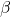–dynamical systems. Mathematika 62 (2016) 884–897. (with M. Coons and Bao-Wei Wang).Preprint.
23. A Jarnik type theorem for planar curves: everything about the parabola. Math. Proc. Camb. Phil. Soc. (2015), 159, 47–60 or download a preprint pdf.
24. A converse to linear independence criteria, valid almost everywhere. Ramanujan J (2015) 38:513–528. (with S. Fischler, S. Kristensen and J. Levesley).
25. A note on the weighted Khintchine–Groshev theorem, Journal de Theorie des Nombres de Bordeaux, 26(2014), 385-397. (with T. Yusupova).
26. The metrical theory of simultaneously small linear forms, Funct. Approx. Comment. Math. 48(2013), No. 2, 167-181. (with J. Levesley).
27. Metrical results on systems of small linear forms, Int. J. of Number Theory 09(2013), No. 03, 769-782. (with S. Kristensen).
28. On weighted inhomogeneous Diophantine approximation on planar curves, Math. Proc. Camb. Phil. Soc. (2013), 154, 225-241. (with T. Yusupova)
29. Badly approximable systems of linear forms in absolute value, Uniform Distribution Theory 8 (2013), no.1, 7-15. (with S. Kristensen).
30. The metric theory of mixed type linear forms, Int. J. of Number Theory,9 (2013), 77-90. (with D. Dickinson).
31. A note on badly approximable linear forms, Bull. Aust. Math. Soc. 83 (2011), 262-266.
32. On fully fuzzy idempotent near-rings, Southeast Asian Bull. of Math. 34(2010), no. 5, 959-970. (with M. Shabir).
33. Fully fuzzy idempotent gamma near-rings, Int. J. Math. Anal.3 (2006), no. 1, 19-37. (with M. Shabir and R.S. Tariq).

Research Statement

Within analytical number theoretic research, I often work within “metric Diophantine approximation and its applications”. The theory of metric Diophantine approximation concerns the approximation properties of real numbers by rationals from a measure-theoretic (probabilistic) point of view. This broad and topical area combines ideas from number theory, measure theory, and dynamical systems. The fundamental question in the theory of Diophantine approximation is, “how well can a real number be approximated by rationals?”. The irrational numbers are uncountable and hence too large to study each individual number one at a time. Consequently, we often group them in classes according to their approximation properties by rational numbers. The set of irrational numbers are classified broadly into two subsets: the set of well-approximable numbers and the set of badly-approximable numbers.

If a Diophantine set (limsup and liminf) of interest has  Lebesgue measure zero (a so-called exceptional set) then one must appeal to a more intricate notion of `size’ to distinguish it from other very small sets. For this reason, related questions of the Hausdorff measure and dimension of exceptional sets arising in Diophantine approximation are subject to much investigation within the field. The main aim of my research is to make significant contributions to the field by establishing a rich metric theory (Lebesgue measure, Hausdorff dimension and Hausdorff measure) concerning a wide class of Diophantine sets.   Given an irrational number, the quality of an approximationis measured in two ways: the error “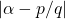”, and the “height”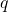. The accuracy and the height are connected with each other so that the error of approximations tends to zero whereas the heights must tend to infinity. Diophantine approximation is about quantifying this tradeoff and describing how it varies over irrational numbers. Once the fundamental  settings are clear, one begins to modify it by extending it to higher dimensions,  by considering other fields such asor number fields and considering more intricate questions such as studying them over manifolds and fractals.

Classical Examples. A consequence of Dirichlet’s theorem (1842) states that any irrational number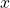can be approximated by infinitely many rationalswith an error of approximation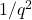. Replacing the error of approximation with a function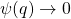as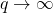raises the question of the size of the set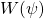of all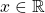such that the inequality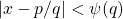is satisfied for infinitely many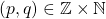. Khintchine’s theorem (1924) asserts that the Lebesgue measure of this set is either zero or full if the sum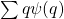converges or diverges respectively. Notice that the Lebesgue measure is zero (oris a null set) for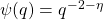for any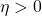, and Khintchine’s theorem gives no further information about the set. To distinguish between the null sets, Hausdorff measure and dimension are the appropriate tools, for example, the Hausdorff dimension of the set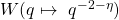~is~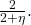On the other hand, the set of badly approximable numbers are defined as the set of irrational numbers for which the error of approximationarising from Dirichlet’s theorem cannot be improved by an arbitrary constant, that is,  there exists a constantsuch that the inequality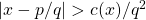is satisfied for all rationals.

The setis an example of a general class of limsup sets and the set of badly approximable numbers is an example of liminf sets. Both of these sets arises naturally in several branches of mathematics. For instance, limsup sets arise in the theory of dynamical systems such as the dynamical system of continued fractions, beta approximations, conformal iterated function systems etc as the so-called shrinking target and recurrence sets.  To be precise, let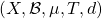be a metric measure-preserving system (m.m.p.s.), by which we mean that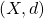is a metric space,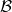is a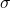-field containing the Borel-field of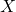, and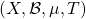is a measure-preserving dynamical system.

We unify the recurrence and shrinking target problems by a sequence of Lipschitz functions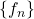.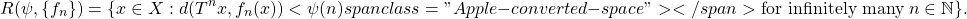The set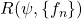is another example of a limsup set. In full generality, the size of this set is still unknown.

Long term Research Goals.  My long-term research goals are to develop new powerful measure theoretic techniques in mathematics that will be used in establishing some indispensable results in analytical number theory (Diophantine approximation) and dynamical systems. In particular, the new techniques will help in estimating the sizes of limsup and liminf sets such as those stated above in various settings. The plan is to construct new techniques and to use them in situations where existing techniques are not applicable. As a consequence, I envisage that the new techniques will have far-reaching applications beyond the confines of Diophantine approximation and dynamical systems, for example, geometric measure theory, fractal analysis, geometric probability, and stochastic geometry etc.

Research contributions to the field

Below I list some of my significant contributions to the field. Note that most of the journals (below) are considered generalist mathematics journals which put an additional emphasis on the results to appeal to diverse mathematics readership.

1. Diophantine Approximation on Manifolds. Papers [6, 11, 16, 27, 33] are in this direction of research.
2. Diophantine approximation and Metric Geometry. Papers [9, 15, 19, 22, 24] are in this direction of research.
3. Shrinking Target and Recurrence Theory. Papers [12, 18, 31, 32] are in this direction of research.
4. The Absolute Value Theory. Papers [3, 4, 5, 7, 8, 10, 17, 20, 23, 24] are in this direction of research.
5. Dirichlet Improvability. Papers [ 21, 25, 26, 29, 30] are in this direction of research.